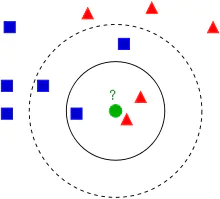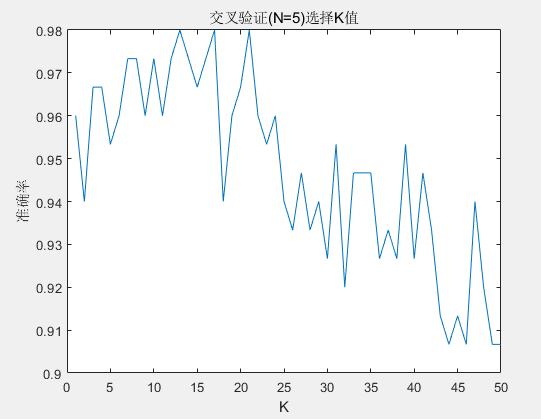• matlab自带knn函数
2021-04-18 10:37:33

话说matlab的模式识别工具箱非常强大，对于一般应用来说基本不用自己编程，这就给科研带来了极大的便利。

之后估计会利用这个工具箱里的许多分类方法，比如KNN、BP、SVM等等；

现在就利用knn来进行分类。

For the object

of samplify, I only choose 3 classes while 2 samples for each class

,there are 18 features in every sample

.

准备条件：已经把特征数据和样本标号保存为文件。

测试代码为：

1.

2.

3.

4.

k=knnclassify(test_data,train_data,train_label,3,'cosine','random');

train_data保存的是训练样本特征，要求是最能代表本类别的，不一定多，当然不能太少；

train_label保存的是样本标号，如0,1,2等等，随便设置，只有能区分就行,具体格式可以为:

1.

1 1 2 2 3 3

test_data测试文件保存的是测试数据的特征；

关键函数介绍：

knnclassify是利用最近邻进行分类的分类器；

函数调用形式：

1.CLASS =

KNNCLASSIFY(SAMPLE,TRAINING,GROUP)

标号和训练数据必须有相同的行数；训练数据和测试数据必须有相同的列；函数对于无效值或者空值会作为丢失值或者忽略这一行。

2.CLASS =

KNNCLASSIFY(SAMPLE,TRAINING,GROUP,K)

此函数允许你设置距离矩阵形式，如：

'euclidean'欧氏距离，默认的

'cityblock'绝对差的和

'cosine'角度距离

'correlation'相关距离

'Hamming'汉明距离

3.CLASS

=KNNCLASSIFY(SAMPLE,TRAINING,GROUP,K,DISTANCE,RULE)

本函数允许你选择如何对样本进行分类，如你可以选择：

'nearest'最近的K个的最多数

'random'随机的最多数

'consensus'投票法，默认的

而我选择的函数形式是：

k=knnclassify(test_data,train_data,train_label,3,'cosine','random');

大家可以对照看看选择合适的，多试试。

嗯，很简单，就这样就可以得到最后的分类类别了。

对了，还要把最后的分类类别进行保存，之前的一篇已经提到如何保存了，最终代码：

1.

2.

3.

4.

k=knnclassify(test_data,train_data,train_label,3,'cosine','random');

5.

dlmwrite('a.txt',b,'delimiter',' ','newline','pc');

更多相关内容
• 优化knn代码matlab ############################################### ################### PROJECT 2: COMP 551 - LANGUAGE CLASSIFICATION author: AMAR KUMAR[amar.kumar@mail.mcgill.ca] LITA FAN [lita.fan...
• 模式识别中的KNN算法实现，基于Matlab的实现，以及剪辑近邻法的matlab实现。
• 利用KNN算法对sonar数据分类，用Matlab语言实现
• 高光谱分类knn matlab代码FNGBS 这是用于高光谱波段选择的快速邻域分组方法的实现。 数据集 四个公共数据集，即 、 、 、 和 ，被用来验证所提出的 FNGBS 的有效性。 要求 MATLAB、libsvm、cruve拟合工具 执行 关于 ...
• matlab knn源代码KNN_分类 支持 MPI 和 openMP 的 KNN 分类 程序的源代码位于Source文件夹中。 有3个版本： MPI 阻塞版本MPI_B_KNN.c MPI 非阻塞版本MPI_NB_KNN.c 串行版本Serial_KNN.c 可以在此处找到用于生成在...
• 划分数据集：将64组数据作为训练数据，16组数据作为测试数据，4类信号在训练集和测试集的数量比例相同，将训练集和测试集整体归一化，再分别作为KNN的输入。 源程序是利用KNN算法对训练集和测试集整体归一化后的测试...
• matlab 10折交叉验证知识代码KNN算法方法 针对数据挖掘课程设计了不同的KNN算法。 有一个与患者的癌症状况相关的数据集，具有10个不同的特征，并且是用于诊断的类部分。 “ 1”用于诊断疾病，“ 0”反之亦然。 在...
• knn基于matlab的代码PIFW-kNNMATLAB实现。 撰写者：Nimagna Biswas和Saurajit Chakraborty。 参考：Nimagna Biswas，Saurajit Chakraborty，Sankha Subhra Mullick和Swagatam Das，参数独立模糊加权k最近邻分类器...
• 简单的K近邻算法，这里用于分类，已经调试了，加了注释，方便大家看
• 这里的文件是： (1) load_data：从face_images.mat和nonface_images.mat加载数据face_images.mat 文件应包含： - train_imgs：包含 N 个测试人脸图像的 NxMxL 张量。 每个图像都是 MxL 像素（灰度）。...
• ## knnmatlab

热门讨论 2009-08-15 10:13:00
Efficient K-Nearest Neighbor Search using JIT knn 算法matlab实现 测试版本：MATLAB 7.5 (R2007b)
• 很有用 可运行 KNN学习算法实现，演示回归算法算法在计算机视觉中的应用 ，实现如何利用偏最小二乘回归模型实现数据拟合
• matlab实现的KNN分类的源代码，十分简单易用
• ## knn算法matlab实现

热门讨论 2011-11-15 10:06:57
knn算法matlab实现，较简单，大家多指导指导
• 优化knn代码matlab CogSciClass 中的机器学习 为机器学习任务开发的 Matlab 脚本认知科学机器学习课程。 KNN_kitchell 使用多数投票或距离加权在一组点上运行 K 最近邻的脚本 均值偏移 用于应用 K-means 或 Mean ...
• ## KNN算法Matlab实现

千次阅读 2021-10-14 17:02:40
KNN算法Matlab实现 简介： KNN全称K-Nearest Neighbors,源于20世纪70年代。属于有监督学习的传统机器学习算法，既可以解决回归问题也可解决分类问题。

# KNN算法Matlab实现

### 简介：

KNN全称K-Nearest Neighbors,源于20世纪70年代。属于有监督学习的传统机器学习算法，既可以解决回归问题也可解决分类问题。本文章只研究K-NN的分类问题，其核心思想是离样本A距离最近的K个样本的类别代表了A的类别。放一个经典案例图：在这个问题中，需要考虑三个核心要素：1）K值如何得到 2）样本间的距离如何定义 3）投票机制。

### K值如何得到

答案是K折交叉验证法，此算法就是任意将训练数据集切分为K份，（K-1）份作为训练数据集，1份用于性能评估，其常被用到机器学习模型超参数调优上，此不详细赘述。

### 样本间的距离如何定义

包括但不限于如下：

• 曼哈顿距离
d 12 = ∑ k = 1 n ∣ x 1 k − x 2 k ∣ d_{12}=\sum^n_{k=1}|x_{1k}-x_{2k}|
• 欧几里得距离
d 12 = ∑ k = 1 n ∣ x 1 k − x 2 k ∣ d_{12}=\sqrt{\sum^n_{k=1}|x_{1k}-x_{2k}|}
• 马氏距离
D ( X i , X j ) = ( X i − X j ) T S − 1 ( X i − X j ) D(X_i,X_j)=\sqrt{(X_i-X_j)^TS^{-1}(X_i-X_j)}
• 闵可夫斯基距离
d 12 = ∑ k = 1 n ∣ x 1 k − x 2 k ∣ p p d_{12}=\sqrt[p]{\sum^n_{k=1}|x_{1k}-x_{2k}|^p}
最常用的是欧氏距离

### 投票机制

1. 少数服从多数
2. 距离加权投票（最常用）

### 代码实现

选用iris数据集作为训练测试的数据集，距离使用欧氏距离，投票机制使用少数服从多数。

iris=xlsread("iris.csv");
iris=iris(:,2:6);
K_Max=50;
acc_avg_history=[];
for k=1:K_Max
%K交叉验证
N=5;%K交叉验证的份数
rawrank=randperm(size(iris,1));%打乱顺序
rand_iris=iris(rawrank,:);
cell_iris=mat2cell(rand_iris,150/N*ones(1,N));%分为N份
for n=1:N
cell_iris=circshift(cell_iris,1);%移位一次
train_set=cell2mat(cell_iris(1:N-1));%取N-1份做训练集
test_set=cell_iris{N};%取N份做测试集
pre_label=knn(test_set(:,1:4),train_set,k);%KNN预测花卉种类
accuracy(n)=sum(pre_label==test_set(:,5))/(150/N);%求正确率
end
acc_average=mean(accuracy);%求平均正确率
acc_avg_history=[acc_avg_history acc_average];%保存不同K值下的正确率
end
plot(acc_avg_history);xlabel('K');ylabel('准确率');title('交叉验证(N=5)选择K值')
function out=knn(test_set,train_set,K)
[n ,~]=size(test_set);
[m,~]=size(train_set);
for i=1:n
for j=1:m
%求距离
distance(j)=sqrt(sum((test_set(i,:)-train_set(j,1:4)).^2));
end
[~,index]=sort(distance,'ascend');
label=train_set(index,5);%按照距离大小排序
out(i)=mode(label(1:K));%取前K个标签里出现次数最多的那个标签
end
out=out';
end


运行结果：不同K值对应的准确率机器学习观止核心原理与实践，林学森著

展开全文算法 机器学习
• 可以输出概率的KNN分类器matlab源程序，带注释
• 1.算法流程1)通过Matlab产生高斯分布产生两类数据，并标明类别2)数据初始化：设置K=某个常数(一般为奇数)3)对于每个测试数据，计算其到两类数据的所有点的距离对于上述求得的距离，选出K个最小的，检测这选出的K个值...

1.     算法流程

1)      通过Matlab产生高斯分布产生两类数据，并标明类别

2)      数据初始化：设置K=某个常数(一般为奇数)

3)      对于每个测试数据，

计算其到两类数据的所有点的距离

对于上述求得的距离，选出K个最小的，

检测这选出的K个值中属于类别1和类别2的个数

If Cnt1>Cnt2

属于类别1；

Else

属于类别2；

2、实验代码：

clear all;

close all;

clc;

%%第一个类数据和标号

mu1=[0 0];  %均值

S1=[0.3 0;0 0.35];  %协方差

data1=mvnrnd(mu1,S1,100);   %产生高斯分布数据

plot(data1(:,1),data1(:,2),'+');

label1=ones(100,1); %产生100行1列的单位矩阵

hold on;

%%第二个类数据和标号

mu2=[1.25 1.25];

S2=[0.3 0;0 0.35];

data2=mvnrnd(mu2,S2,100);

plot(data2(:,1),data2(:,2),'ro');

label2=label1+1; %产生100行1列的全为2的矩阵

data=[data1;data2];%数据合并

label=[label1;label2];

grid on;

K=11;   %两个类，K取奇数才能够区分测试数据属于那个类

%测试数据，KNN算法看这个数属于哪个类

for ii=-3:0.1:3

for jj=-3:0.1:3

test_data=[ii jj];  %测试数据

label=[label1;label2];

%%下面开始KNN算法，显然这里是11NN。

%求测试数据和类中每个数据的距离，欧式距离(或马氏距离)

distance=zeros(200,1); %产生200行1列的零矩阵

for i=1:200

distance(i)=sqrt((test_data(1)-data(i,1)).^2+(test_data(2)-data(i,2)).^2); %(xi-X)2+(yj-Y)2

end

%选择排序法，只找出最小的前K个数据,对数据和标号都进行排序

for i=1:K

ma=distance(i);

for j=i+1:200

if distance(j)

ma=distance(j);

label_ma=label(j);

tmp=j;

end

end

distance(tmp)=distance(i);  %排数据

distance(i)=ma;

label(tmp)=label(i);        %排标号，主要使用标号

label(i)=label_ma;

end

cls1=0; %统计类1中距离测试数据最近的个数

for i=1:K

if label(i)==1

cls1=cls1+1;

end

end

cls2=K-cls1;    %类2中距离测试数据最近的个数

if cls1>cls2

plot(ii,jj);     %属于类1的数据画小黑点

end

end

end

3、结果说明

本算法的测试数据是坐标轴上的点，范围是x[-3,3], y[-3,3];

最终结果一类用黑点表示，一类用空白表示

4、算法结果：展开全文• 直接使用KNN，将自己的数据集直接代入到KNN中，可以分类出自己想要的结果
• KNNmatlab源程序，自己为近红外实验数据处理的
• 将其进行改进，代码更加简练，并使用数据进行测试： KNN.m: function [ idx ] = KNN( trainData,trainClass,testData,K ) %UNTITLED Summary of this function goes here % Detailed explanation goes here [N,M]=...

clear;

clc;

K=3;

trainData = [1.0,2.0;1.2,0.1;0.1,1.4;0.3,3.5];

trainClass = [1,1,2,2];

testData = [0.5,2.3];

[N,M]=size(trainData);

%计算训练数据集与测试数据之间的欧氏距离dist

dist=zeros(N,1);

for i=1:N

dist(i,:)=norm(trainData(i,:)-testData);

end

%将dist从小到大进行排序

[Y,I]=sort(dist,1);

%将训练数据对应的类别与训练数据排序结果对应

trainClass=trainClass(I);

%确定前K个点所在类别的出现频率

classNum=length(unique(trainClass));%取集合中的单值元素的个数

labels=zeros(1,classNum);

for i=1:K

j=trainClass(i);

labels(j)=labels(j)+1;

end

%返回前K个点中出现频率最高的类别作为测试数据的预测分类

[~,idx]=max(labels);

fprintf('该测试数据属于类 %d\n',idx);

将其进行改进，代码更加简练，并使用数据进行测试：

KNN.m:

function [ idx ] = KNN( trainData,trainClass,testData,K )

%UNTITLED Summary of this function goes here

% Detailed explanation goes here

[N,M]=size(trainData);

%计算训练数据集与测试数据之间的欧氏距离dist

dist=zeros(N,1);

for i=1:N

dist(i,:)=norm(trainData(i,:)-testData);

end

%将dist从小到大进行排序

[Y,I]=sort(dist,1);

K=min(K,length(Y));

%将训练数据对应的类别与训练数据排序结果对应

labels=trainClass(I);

%{

%确定前K个点所在类别的出现频率

classNum=length(unique(trainClass));%取集合中的单值元素的个数

labels=zeros(1,classNum);

for i=1:K

j=trainClass(i);

labels(j)=labels(j)+1;

end

%返回前K个点中出现频率最高的类别作为测试数据的预测分类

[~,idx]=max(labels);

%}

%确定前K个点所在类别的出现频率

idx=mode(labels(1:K));%mode函数求众数

fprintf('该测试数据属于类 %d ',idx);

end

KnnDataTest.m:

clear;

clc;

ratio=0.1;%测试数据所占比例

[N,M]=size(X);

K=4;

trainData=X(:,1:3);

trainClass=X(:,4);

num_test=N*ratio;

%归一化处理newData=(oldData-minValue)/(maxValue-minValue);

minValue=min(trainData);

maxValue=max(trainData);

trainData=(trainData-repmat(minValue,N,1))./(repmat(maxValue-minValue,N,1));

error=0;

for i=1:num_test

idx=KNN(trainData(num_test+1:N,:),trainClass(num_test+1:N,:),trainData(i,:),K);

fprintf('该测试数据的真实类为：%d\n',trainClass(i,:));

if idx~=trainClass(i,:);

error=error+1;

end

end

fprintf('准确率为：%f\n',1-error/num_test);

部分结果：展开全文• ## 用matlab实现knn分类

热门讨论 2013-05-26 16:16:41
该算法用matlab实现了knn分类，knn分类是数据挖掘中很经典的算法之一
• ## matlab-knn使用

千次阅读 2021-02-22 19:27:56
knnmatlab实验【使用UCI数据集】3.0. KNN函数自带用例3.1. UCI数据集3.2. knn实验计划3.3. 数据集筛选3.4. play with knn4. 总结 1. 什么是knn 1.1. 什么是knn 来了一个无标签的样本，找它的周围邻居（有标签）...机器学习
• KNN函数源码 包括主函数cvKnn，和被调用函数cvEucdist和constructW 主函数cvKnn: % cvKnn - K-Nearest Neighbor classification % % Synopsis % [Class] = cvKnn(X, Proto, ProtoClass, [K], [distFunc]) % % ...分类 矩阵
• 一、KNN算法原理 1.算法概述 k近邻(k-Nearest Neighbor，简称kNN)学习是一种常用的监督学习方法，其工作机制非常简单：给定测试样本，基于某种距离度量找出训练集中与其最靠近的k个训练样本，然后基于这k个"邻居...机器学习 算法
• 关于数据集神马的，请直接参考：http://blog..net/wangyuquanliuli/article/details/11606435这里直接给出KNN matlab的实现trainImages = loadMNISTImages('train-images.idx3-ubyte');trainLabels = ......

# knn matlabmatlab 订阅